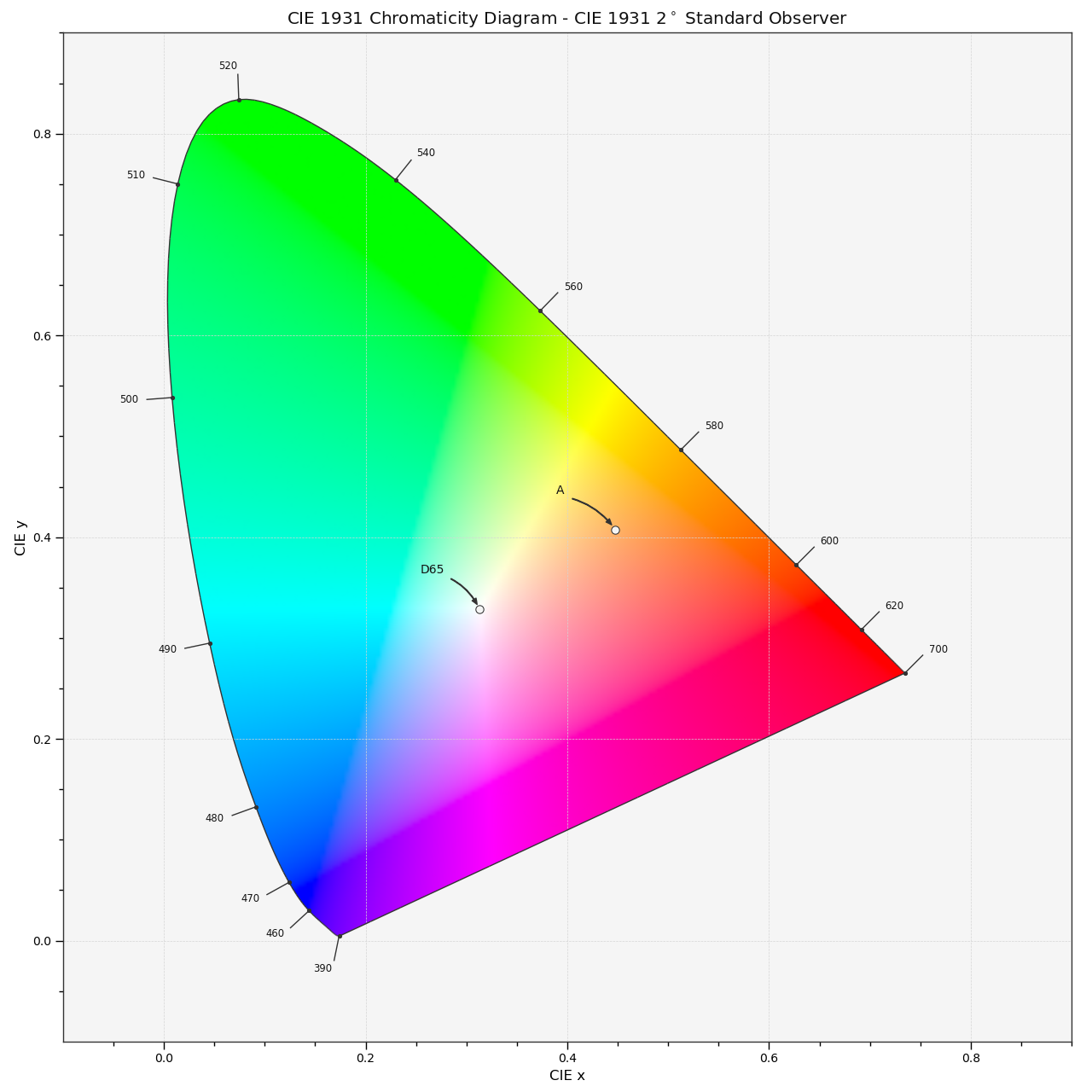# colour.plotting.plot_sds_in_chromaticity_diagram_CIE1931¶

colour.plotting.plot_sds_in_chromaticity_diagram_CIE1931(sds, cmfs='CIE 1931 2 Degree Standard Observer', annotate_parameters=None, chromaticity_diagram_callable_CIE1931=<function plot_chromaticity_diagram_CIE1931>, **kwargs)[source]

Plots given spectral distribution chromaticity coordinates into the CIE 1931 Chromaticity Diagram.

Parameters: Other Parameters: sds (array_like, optional) – Spectral distributions to plot. cmfs (unicode, optional) – Standard observer colour matching functions used for Chromaticity Diagram bounds. annotate_parameters (dict or array_like, optional) – Parameters for the plt.annotate() definition, used to annotate the resulting chromaticity coordinates with their respective spectral distribution names if annotate is set to True. annotate_parameters can be either a single dictionary applied to all the arrows with same settings or a sequence of dictionaries with different settings for each spectral distribution. chromaticity_diagram_callable_CIE1931 (callable, optional) – Callable responsible for drawing the CIE 1931 Chromaticity Diagram. **kwargs (dict, optional) – {colour.plotting.artist(), colour.plotting.diagrams.plot_chromaticity_diagram(), colour.plotting.render()}, Please refer to the documentation of the previously listed definitions. Current figure and axes. tuple

Examples

>>> from colour import ILLUMINANTS_SDS
>>> A = ILLUMINANTS_SDS['A']
>>> D65 = ILLUMINANTS_SDS['D65']
>>> plot_sds_in_chromaticity_diagram_CIE1931([A, D65])  # doctest: +SKIP